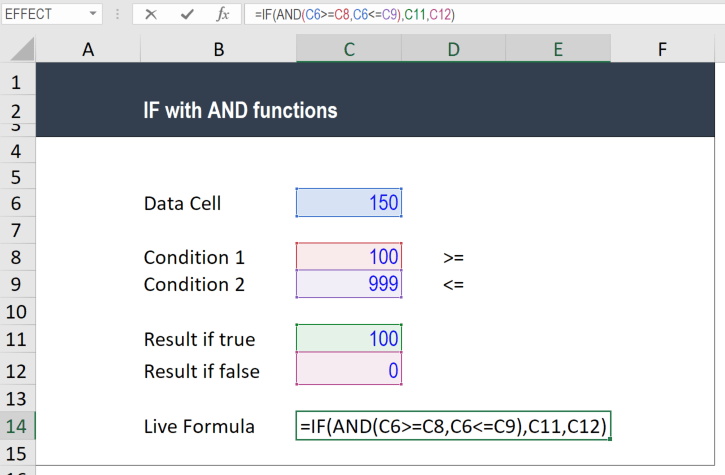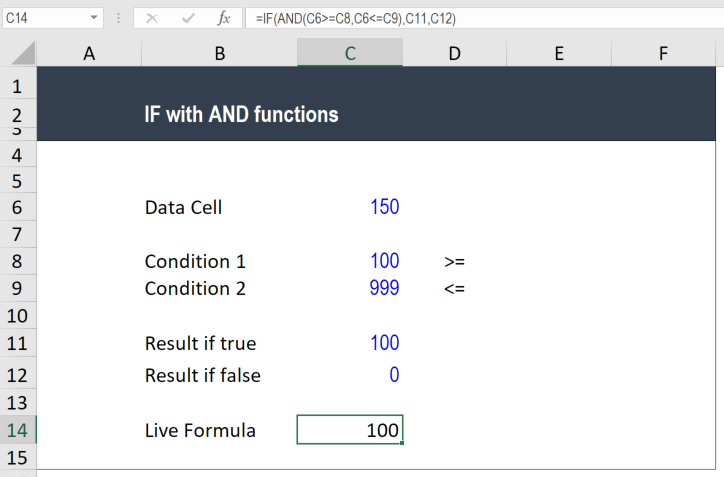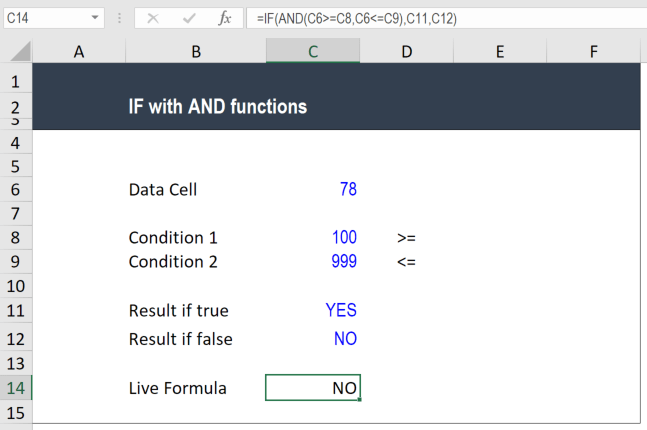# IF Statement Between Two Numbers

This article outlines how to use the IF with AND functions in Excel

## How to Combine IF with AND functions

In Excel, you can combine IF with AND functions to return a value based on two different numbers. It can be very useful when performing financial modeling and when you are creating conditional situations. In this article, learn how to build an IF statement between two numbers so you can easily answer the problem you’re trying to solve.

For example, if you are looking for a formula that will go into cell B2 and, if the number is between 100 and 999, then the result will be 100. Otherwise, if it is outside that range, then the result will be zero.

### IF statement between two numbers

=IF(AND(C6>=C8,C6<=C9),C11,C12)

(See screenshots below).

Example of how to use the formula:

Step 1: Put the number you want to test in cell C6 (150).

Step 2: Put the criteria in cells C8 and C9 (100 and 999).

Step 3: Put the results if true or false in cells C11 and C12 (100 and 0).

Step 4: Type the formula =IF(AND(C6>=C8,C6<=C9),C11,C12).### Final result

Here is a screenshot in Excel after using the formula for an IF statement between two numbers. You can clearly see how the result from the example is 100 because the number 150 is between 100 and 999.Congratulations, you have now combined IF with AND between two numbers in Excel!

### IF statement between two numbers (with text)

You don’t have to limit the resulting output from the model to only numbers. You can also use text, as shown in the example below.  This time, instead of producing 100 or 0 as the result, Excel can display YES or NO to show if the argument is true or false.### More Excel Tutorials

Thank you for reading this guide to understanding how to use the IF function with the AND function in Excel to know if a number is between two other numbers. To keep learning and developing your career as a financial analyst, these additional CFI resources will help you on your way:

• Index and Match Functions
• Excel Shortcuts List
• Important Excel Formulas